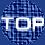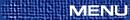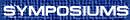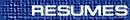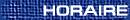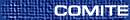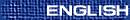Abstract Harmonic Analysis / Analyse harmonique abstraite
(Anthony Lau and Keith Taylor, Organizers)

An abstract formulation of wavelet theory

We present an abstract formulation for an ``internal affine structure'' on a Hilbert space in terms of a unitary representation of a special kind of group. Given such an affine structure, natural definitions of wavelet subspace and multiresolution analyses can easily be made. Some of the ``magical'' formulas of wavelet theory are clarified from this abstract point of view.

BRIAN FORREST, Department of Pure Mathematics, University of Waterloo, Waterloo, Ontario  N2L 3G1
The Fourier algebra as an operator space

As the predual of a von Neumann algebra, the Fourier algebra A(G) of a locally compact group G has a natural operator space structure. In this talk we will show how the operator space structure can play an important role in studying the Fourier algebra of a nonabelian group.

JOHN FOURNIER, Department of Mathematics, University of British Columbia, Vancouver, British Columbia  V6T 1Z2
The peculiar cases of the lower majorant property

Use the symbol [^(f)](n) for the coefficients in the complex form of the Fourier series of a function f on the interval [-p, p). It is known that if p = 2k/(2k-1) for some positive integer k > 1, then for each function f in Lp there exists a function F in Lp that majorizes f in the sense that [^(F)](n) ³ |[^(f)](n)| for all n, but that also satisfies ||F||p £ ||f||p. The existence proofs for such paradoxical majorants do not provide constructions of them, but each function in one of these peculiar Lp spaces has a unique majorant of minimal Lp-norm. We add slightly to the classical existence proof and a modern proof, and

get some interesting restrictions on the form of the minimal majorant. We also do this for the Schatten norms on matrices.

FEREIDOUN GHAHRAMANI, Department of Mathematics, University of Manitoba, Winnipeg, Manitoba  R3T 2N2
Point derivations and amenability of measure algebras

Let G be a locally compact group. In 1972 B. E. Johnson proved that the group algebra of G is an amenable Banach algebra if and only if G is an amenable group. The question of amenability of the measure algebras was left open. In 1976 G. Brown and W.Moran proved that if G is non-discrete and abelian then the measure algebra of G has a non-zero point derivation. We show that the measure algebra of every non-discrete group G has a non-zero point derivation. This in particular resolves the questions about weak amenability and amenability of the measure algebrs. This is joint work with H. G. Dales and A. Ya. Helemskii.

COLIN GRAHAM, Bowen Island
Arens Regularity for quotients Ap(E) of the Herz algebra

If the subset E of T supports a synthesizable p-pseudofunction T, then the restriction Ap(E) to E of the Herz algebra on T is not Arens regular. The proof is direct, by brute force, and does not show the existence of Day points (see below) for Ap(E).

EDMOND GRANIRER, Department of Mathematics, University of British Columbia, Vancouver, British Columbia  V6T 1Z2
A few remarks on quotients of the Fourier Algebra

Some functional analytic properties of quotients of the Fourier and the Herz algebras connected with the nonuniqueness of invariant means on the space of pseudomeasures will be discussed.

ZHIGUO HU, University of Windsor, Windsor, Ontario  N9B 3P4
Inductive extreme non-Arens regularity of the Fourier algebra

Let G be a locally compact group and let A(G) be the Fourier algebra of G. In this talk, we will show that A(G) is inductive extreme non-Arens regular in the sense that A(G) is an inductive union of a family of Fourier algebras such that this family has an inductively compatible extreme non-Arens regularity.

WOJCIECH JAWORSKI, School of Mathematics and Statistics, Carleton University, Ottawa, Ontario  K1S 5B6
Probability measures on almost connected amenable locally compact groups and some related ideals in group algebras

Given a locally compact group G let Ja(G) denote the set of all closed left ideals J in L1(G) which have the form J=[L1(G)*(de -m)]- where m is an absolutely continuous probability measure on G. We explore the order structure of Ja(G) when Ja(G) is ordered by inclusion. When G is connected and amenable, every nonempty family F Í Ja(G) admits both a minimal and a maximal element, in particular, every ideal in Ja(G) contains an ideal that is minimal in Ja(G). Furthermore, every chain in Ja(G) is necessarily finite. A natural generalization of these results to almost connected amenable groups is discussed. Our proofs use results from the theory of boundaries of random walks.

Hardy's uncertainty principle for Lie groups

Roughly speaking, the classical uncertainty principles say that a nonzero function on the real line and its Fourier transform cannot simultaneously be concentrated. One possibility of measuring concentration is to consider the decay of a function at infinity. An important result making this precise is Hardy's theorem (1933). Naturally, there has been some effort to prove Hardy like theorems for various connected Lie groups. Specifically, analogues of Hardy's theorem have been shown for the Heisenberg groups, for Euclidean motion groups, for Cartan motion groups and for SL(2,R). Very recently and most notably, analogues of Hardy's uncertainty principle were established for general non-compact connected semi-simple Lie groups with finite centre and for arbitrary connected and simply connected nilpotent Lie groups. In all these cases the decay condition for the Fourier transform has to be replaced by a similar condition on the Hilbert-Schmidt norm of the operator valued Fourier transform on the dual space of the underlying group. The talk will present some of these results and will focus on explaining the set-up.

Regular representations of groupoids

The left regular representation of a locally compact groupoid is constructed in the Hausdorff as well as in the non-Haudorff case. In the Hausdorff case, this representation takes place on a natural Hilber module over C0(X) where X is the space of units of the groupoid. In the non-Hausdorff case, a natural locally compact space Y, which contains X as a dense subset, is constructed. The representation takes place on a Hilber C0(Y)-module.

LAURENT MARCOUX, University of Alberta, Edmonton, Alberta
Weak amenability of triangular Banach algebras

Let A and B be unital Banach algebras and M be a Banach A, B-module. Then T = [

 A
 M
 0
 B
] becomes a triangular Banach algebra when equipped with an appropriate norm. A Banach algebra is said to be n-weakly amenable if all derivations from that algebra into its n-th dual space are inner. In this talk we investigate the n-weak amenability of triangular Banach algebras in terms of the n-weak amenability of A and B and their action upon M.

TIANXUAN MIAO, Department of Mathematics, Lakehead University, Thunder Bay, Ontario  P7B 5E1
On the multiplier algebra of Ap(G)

Let BpM(G) be the pointwise multiplier algebra of the Figa-Talamanca-Herz algebra Ap(G) equipped with the multiplier norm on BpM(G). We will prove that BpM(G) is a dual Banach space. Properties of BpM(G) such as the density of Ap(G) in BpM(G) will be discussed.

MATTHIAS NEUFANG, Department of Mathematical Sciences, Edmonton, Alberta  T6G 2G1
Abstract harmonic analysis and quantized functional analysis

Among the most interesting objects in abstract harmonic analysis are the measure algebra M(G) and the dual LUC(G)* of the bounded left uniformly continuous functions on a locally compact group G, endowed with the Arens product. Adopting the point of view of operator spaces, we present the construction of an isometric representation of these algebras in the algebra CB(B(H)) of completely bounded operators on B(H), where H=L2(G), which extends earlier work of E. Størmer and F. Ghahramani.

The talk gives an overview of the various fruitful interactions between abstract harmonic analysis and quantized functional analysis in this framework. The central features are operator analogues of the well-known theorems of Brainerd/Edwards and Curtis/Figà-Talamanca. Further investigating the structure of the image algebras arising from our representation, we obtain bicommutant theorems for the latter which parallel von Neumann's classical bicommutant theorem in the setting of completely bounded operators on B(H).

Our main tools are factorization theorems for families in the left LUC(G)*-modules LUC(G), L¥(G) and B(L2(G)), respectively, that are of interest on their own right. These yield results about automatic normality, on the one hand, thus moreover providing a completely new approach to the topological center problem for L¥(G)* and LUC(G)* (which is closely related to the (bi)commutant problems mentioned above), and on automatic continuity, on the other hand, answering in particular a question raised by G. Wittstock in 1997.

VOLKER RUNDE, University of Alberta, Edmonton, Alberta
Operator Figà-Talamanca-Herz algebras

Let G be a locally compact group. We use the canonical operator space structure on the spaces Lp(G) for p Î [1,¥] introduced by G. Pisier to define operator space analogues OAp(G) of the classical Figà-Talamanca-Herz algebras Ap(G). If p Î (1,¥) is arbitrary, then Ap(G) Ì OAp(G) such that the inclusion is a contraction; if p = 2, then OA2(G) @ A(G) as Banach spaces spaces and, for amenable G, the identity from A(G) to OA2(G) is a complete contraction. We show that OAp(G) is a completely contractive Banach algebra for each p Î (1,¥), and that OAq(G) Ì OAp(G) completely contractively for amenable G if 1 < p £ q £ 2 or 2 £ q £ p < ¥. Finally, we characterize the amenability of G in terms of the operator amenability of OAp(G) for arbitrary p Î (1,¥), thus extending a result by Zh.-J. Ruan for the Fourier algebra A(G).

ECKART SCHULZ, Suranaree University of Technology, Nakhon Ratchasima, Thailand
Continuous and semi-discrete tight frames on Rn

Given A Î GLn(R), we show that there exists a function w Î L2(R) whose dilates by powers of A and translates form a semi-discrete frame on L2(Rn) if and only if |det(A)| ¹ 1. If in addition, A lies in the exponential group of GLn(R) then there exists w Î L2(Rn) whose dilates by exponents of A and translates form a continuous frame on L2(Rn) if and only if det(A) ¹ 1.

PETER WOOD, Wilfrid Laurier University
Invariant complementation and projectivity in A(G)

In the category of operator spaces, it is known that the Fourier algebra A(G) is amenable if and only if G is amenable. Amenability is a powerful property which, for our purposes, allows us to perform ``averaging arguments'' which might not otherwise be available.

For example, let I be a closed ideal in the Fourier algebra A(G). In this talk we investigate when I is complemented. The general approach to this question has been to construct projections onto I which are invariant, i.e. we find P: A(G)® I such that P(ax)=aP(x) for all a, x Î A(G). Once such a projection is constructed, reasonable necessary conditions on I can be determined.

Historically, such projections were constructed by utilizing the amenability of the algebra. However, the language of homological algebra, amenability becomes one of many ``invariance'' type properties. Another important such property is biprojectivity.

In this talk, we shall show that A(G) is biprojective in the category of operator spaces if and only if G is discrete. Using this result, we can show that for discrete groups, every complemented ideal I of A(G) is complemented by an invariant projection.

YONG ZHANG, Department of Mathematics, University of Manitoba, Winnipeg, Manitoba  R3T 2N2
Approximate identities for ideals of Segal algebras on a compact group

In the monograph ``L1-Algebras and Segal Algebras'' H. Reiter has shown that every closed ideal of a symmetric Segal algebra on a compact group admits an approximate identity. With a different way of approach we show that every closed ideal of a symmetric or pseudosymmetric Segal algebra on a compact group admits an approximate identity. Moreover, for a compact group G, a closed left (right) ideal J of L1(G) has a right (resp. left) approximate identity (ea) with a*ea (resp. ea*a) converging to a uniformly for a in compact sets of J if and only if J is approximately complemented in L1(G).